# Table of derivatives of elementary functions, derivative of a function

Table of derivativecomputing the derivative, which in turn is the most important operation in differential calculus. Therefore, using the table of derivatives you will be able to quickly solve any problem.

Definition: the Derivative of the functionat the point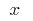is called the limit of the ratio of the increment function at the pointthe increment of the argument, when the increment of the argument tends to zero (can be designatedprime or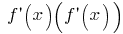## General differentiation formula

Data in the above formulas, u and v are arbitrary differentiable functions and c is a constant. Formulas of differentiation, given below, is sufficient to differentiate any elementary function.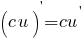(derivative of a constant number)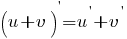(derived amount)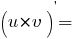(derivative works)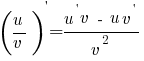(the derived share)

## Table of derivatives of elementary functions

### Derivative of constants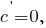where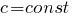### The derivative of the exponential functionDerivative of exponential functions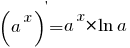### The derivative of the exponent### The derivative of the logarithm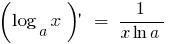### Derivative of the natural logarithm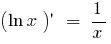### Derivative of cosine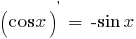### Derivative of sine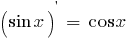### Derivative of tangent### Derivative of cotangent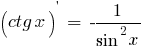### Derivatives of inverse trigonometric functions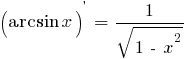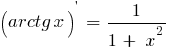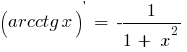### Derivatives of hyperbolic functions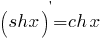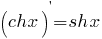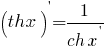Or to find the second derivative

Or is better to understand what is a derivative?

Versions in other languages:
Share with friends: